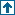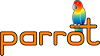parrotcode: MatchRange Numbers PMC Class Contents | Dynamic PMCs

# NAMEclasses/matchrange.pmc - MatchRange Numbers PMC Class

# DESCRIPTION`MatchRange` provides a representation of regular expression matches, by describing the starting and ending offsets within the input string. This PMC only provides the start and end values; the Match PMC is responsible for interpreting these values appropriately (namely, as a substring within the input string.)

This really probably shouldn't be a top-level class; it probably ought to inherit from "IntPair" or something like that. (But it can't just *be* "IntPair", because the Match PMC uses the type to figure out whether it has a regular variable or something that it needs to interpret as a string given its value and the input string.)

This PMC is used by the languages/regex rule compiler, together with the Match PMC.

## Functions`static INTVAL *matchrange_locate_keyed_int(Parrot_Interp interp, PMC *self, STRING *key)`

Interpret the string `key`; valid keys are `start` and `end`, representing the offsets of the first and last characters of the matching range.

## Methods`void class_init()`

Class initialization. Caches the type id of the MatchRange PMC, because it will be used frequently here.

*/

```    void class_init() {
/* class_init_code */
if (pass) {
MatchRange_type_id = entry;
}
}```
/*

`void init()`

Initializes the matchrange with [-2,-2].

`void init_pmc (PMC *initializer)`

Initializes the matchrange number with the specified values. (not implemented)

`void destroy ()`

Cleans up.

`PMC *clone ()`

Creates an identical copy of the matchrange number.

`INTVAL get_bool ()`

Returns true if the match range is defined.

`INTVAL get_integer_keyed (PMC *key)`

`INTVAL get_integer_keyed_str (STRING *key)`

`FLOATVAL get_number_keyed (PMC *key)`

`FLOATVAL get_number_keyed_str (STRING *key)`

`PMC *get_pmc_keyed (PMC *key)`

`PMC *get_pmc_keyed_str (STRING *key)`

Returns the requested number (real part for `real` and imaginary for `imag`).

`INTVAL get_integer_keyed_int(INTVAL key)`

Quick hack to emulate get_start() and get_end():

```  key = 0 ... get start offset
key = 1 ... get end offset```
`void set_integer_keyed_int(INTVAL key, INTVAL v)`

Set start or end depending on key

*/

```    INTVAL get_integer_keyed_int(INTVAL key) {
switch (key) {
case 0:
return RANGE_START(SELF);
case 1:
return RANGE_END(SELF);
default:
internal_exception(1, "MatchRange: key must be 0 or 1");
}
return -2;
}

void set_integer_keyed_int(INTVAL key, INTVAL v) {
switch (key) {
case 0:
RANGE_START(SELF) = v;
break;
case 1:
RANGE_END(SELF) = v;
break;
default:
internal_exception(1, "MatchRange: key must be 0 or 1");
}
}
/*```
`void set_pmc (PMC *value)`

if `value` is a MatchRange PMC then the set the range indices to the same values; otherwise throw an exception. This really only makes sense if you're using a MatchRange from the same Match, or at least for the same input string, but I won't worry about that for now.

`void set_integer_keyed (PMC *key, INTVAL value)`

`void set_integer_keyed_str (STRING *key, INTVAL value)`

`void set_number_keyed (PMC *key, FLOATVAL value)`

`void set_number_keyed_str (STRING *key, FLOATVAL value)`

`void set_number_keyed_int (INTVAL key, FLOATVAL value)`

`void set_pmc_keyed (PMC *key, PMC *value)`

`void set_pmc_keyed_str (STRING *key, PMC *value)`

Sets the requested number (start offset for `start` and end offset for `end`) to `value`. For the keyed_int variants, 0 means RANGE_START, 1 means RANGE_END.

`void add (PMC *value, PMC *dest)`

`void add_int (INTVAL value, PMC *dest)`

`void add_float (FLOATVAL value, PMC *dest)`

Adds `value` to the ending offset of the match range, placing the result in `dest`.

`void subtract (PMC *value, PMC *dest)`

`void subtract_int (INTVAL value, PMC *dest)`

`void subtract_float (FLOATVAL value, PMC *dest)`

Subtracts `value` from the ending offset of the match range, placing the result in `dest`.

`INTVAL is_equal (PMC *value)`

Compares the matchrange number with `value` and returs true if they are equal.